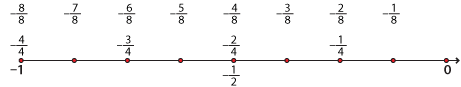### Calculating equivalent fractions

As we saw above,  $$\dfrac{1}{2}$$ is equivalent to $$\dfrac{3}{6}$$.Detailed description

There are two steps of length $$\dfrac{1}{2}$$ to go from 0 to 1.

There are six steps of length  $$\dfrac{1}{6}$$ to go from 0 to 1.

There is one step of length $$\dfrac{1}{2}$$ to go from 0 to $$\dfrac{1}{2}$$.

There are three steps of length $$\dfrac{1}{6}$$to go from 0 to $$\dfrac{1}{2}$$.

We can see that the numerator of $$\dfrac{1}{2}$$ is multiplied by three and the denominator is multiplied by three.

\begin{align}\dfrac{1}{2}&=\dfrac{1×3}{2×3}\\\\ &=\dfrac{3}{6}\end{align}

We can also see that the numerator of $$\dfrac{3}{6}$$ is divided by three and the denominator is divided by three.

\begin{align}\dfrac{3}{6}&=\dfrac{3÷3}{6÷3}\\\\ &=\dfrac{1}{2}\end{align}

In general, to form equivalent fractions from some given fraction, multiply or divide both the numerator and the denominator of the given fraction by the same (non-zero) whole number.

### Negative fractions and the number line

The process for calculating negative equivalent fractions is the same. The number line below demonstrates that $$–\dfrac{6}{8}=\ –\dfrac{3}{4}, –\dfrac{4}{8}=\ –\dfrac{1}{2}\ \text{and}\ –\dfrac{2}{8}=\ –\dfrac{1}{4}.$$Detailed description